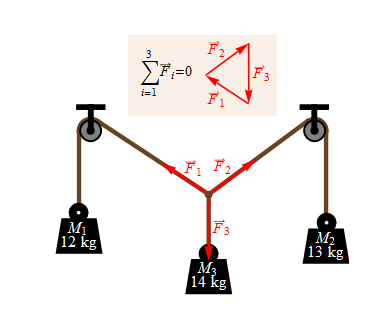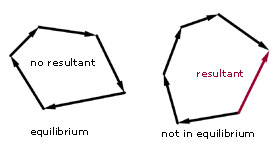## Pages

### 2.3 Forces in equilibrium

Here, we'll touch upon the concepts of forces in equlibrium. If two or more forces acting on a point are in equilibrium, the vector sum of all the forces must be zero. In other words the sum of the components of all the forces must be zero.
$$\sum_{i=1}^{n}F_{i}=0$$
In a special case when there are three coplanar forces say $F_{1},F_{2}$ and $F_{3}$ acting on a point which are in equilibrium, then these forces can be represented in magnitude and direction by the sides of a triangle taken in order as shown in the diagram below.wolframmathematica
The converse is also true that if $3$ coplanar forces acting on a point can be represented in magnitude and direction by the sides of  a triangle taken in order then they must be in equilibrium.

Similarly if there are more than the three forces acts on a point which is in equilibrium then vector triangle is replace by the polygon of forces. If a number of forces are acting at a point, then the missing side in the polygon represents the resultant force. Note the arrow direction on this force is in the opposite direction to the rest.a-levelmathstutor.com
Here's a video on how to draw a vector triangle.

Ex: From the following diagram find the magnitude of $T_{1}, T_{2}$. Use the vector triangle diagram to find the magnitude, without actually doing the calculation.

Sol$^{n}$: $T_{1}=585\pm 15$  and $T_{2}=787\pm15$